1593972060

# Interpreting the model is for humans, not for computers

Critique of pure interpretation
The scientific method as the tool that has served us to find explanations about how things work and make decisions, brought us the biggest challenge that until 2020 we have probably still not overcome: Giving useful narratives to numbers. Also known as “interpretation”.
Just as a matter of clarification, the scientific method is the pipeline of finding evidence to prove or disprove hypotheses. Science and how things work is everything, from natural sciences to economic sciences. But most delightful, by evidence, not only we mean, but humanity understands “data”. And data cannot be anything less than numbers.
Leaving space for generality, the problem of interpretation is particularly entertaining along the path of statistical analyses within the scientific method pipeline. This means finding models that are written in a mathematical language and finding an interpretation for them within the context that delivered the data.
Interpreting a model has two crucial implications that many scientists or science technicians have for long skipped (hopefully not forgotten). The first one relies on the fact that if there is a model to interpret now, there must have been a research question asked before in a context that delivered data to build such a model. The second one is that the narratives we need to create about our model can do much more by expressing ideas about a number within the context of the research question rather than purely inside the model. After all and until 2020, the decisions are made by humans based on the meaning of those numbers, not really by computers. And this last statement is important, because in the 21st century we might actually get to the point that computers take over us in many tasks and they might end up making decisions for us. For this they will need to communicate those decisions among their network. Just then, the human narratives will not count since computers only understand numbers.
As statisticians, we have been adopting the practice of finding problems to solve, finding questions to answer and answers to explain using available data. This mindset has kept us running on a circle of non-sense narratives and interpretations because problems are not found or looked for. Problems and questions emerge from all ongoing interactions and reactions of different phenomena. This fact implies that statistical models and/or other analytical approaches are tools to be used upon the core central problem or question, they are not the spine.
This ugly art of fitting a linear regression on some data and saying that “the beta coefficient is the amount of units that y increases when x increases one unit”, or the art of calculating an average and saying that “it is the value around which we can find the majority of the data points” is a ruthless product that we statisticians have been offering to the scientific method.
The bubble of interpretation
Teaching statistics has made clear for us that people can perfectly understand the way the models work and how to train them to get the numbers. However, what we still did not digest is the fact that out of all the numbers that are produced when training models, most are simply noncommunicable for non statistical people. Let us present some of these numbers whose communication is dark:

• The p-value is one particular concept that may in fact deserve an entire essay on its own. On social media, for instance, we constantly see people asking about an explanation of the p-value and right away there is a storm of statisticians engaging into giving their own interpretation.
• The odds-ratio stars in this debate. After 10 years of working in this field, we must admit that it has never been possible to explain to an expert in another field how to think about the odds ratio. Even Wikipedia has tried out and, in our opinion, has more than failed at it.
• The beta coefficients of a dummy variable in a logistic regression are of the same kind. We have the feeling that they are odds-ratio of the categories with respect to the baseline category. But, how can we make it understandable and actionable in practice? This simply we don’t know.
This problem is central for the scientific and statistical community. The models that we train lose their value because of such a lack of interpretation.
After some years of talking with colleagues about this problem and looking for an appropriate framework that clears up the problem of interpretation, we had come to one obvious conclusion: the interpretation process exists and can only happen within a given context. It makes no sense to fight for the interpretation process inside the model. The inner processes of the model are all numerical and these results can be communicated and understood only by numerical, statistical people. Making interpretations of numbers inside the models is a bubble of rephrasing. In order to interpret the results of a model so that they become tools for taking actions, it is essential to keep in mind the context from where the data is coming and the research questions are asked.

#interpretation #model-interpretability #semantics #data #data-analysis #data analysis

## Buddha Community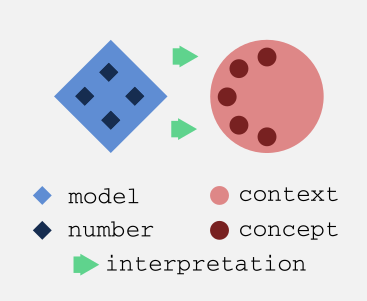1593972060

## Interpreting the model is for humans, not for computers

Critique of pure interpretation
The scientific method as the tool that has served us to find explanations about how things work and make decisions, brought us the biggest challenge that until 2020 we have probably still not overcome: Giving useful narratives to numbers. Also known as “interpretation”.
Just as a matter of clarification, the scientific method is the pipeline of finding evidence to prove or disprove hypotheses. Science and how things work is everything, from natural sciences to economic sciences. But most delightful, by evidence, not only we mean, but humanity understands “data”. And data cannot be anything less than numbers.
Leaving space for generality, the problem of interpretation is particularly entertaining along the path of statistical analyses within the scientific method pipeline. This means finding models that are written in a mathematical language and finding an interpretation for them within the context that delivered the data.
Interpreting a model has two crucial implications that many scientists or science technicians have for long skipped (hopefully not forgotten). The first one relies on the fact that if there is a model to interpret now, there must have been a research question asked before in a context that delivered data to build such a model. The second one is that the narratives we need to create about our model can do much more by expressing ideas about a number within the context of the research question rather than purely inside the model. After all and until 2020, the decisions are made by humans based on the meaning of those numbers, not really by computers. And this last statement is important, because in the 21st century we might actually get to the point that computers take over us in many tasks and they might end up making decisions for us. For this they will need to communicate those decisions among their network. Just then, the human narratives will not count since computers only understand numbers.
As statisticians, we have been adopting the practice of finding problems to solve, finding questions to answer and answers to explain using available data. This mindset has kept us running on a circle of non-sense narratives and interpretations because problems are not found or looked for. Problems and questions emerge from all ongoing interactions and reactions of different phenomena. This fact implies that statistical models and/or other analytical approaches are tools to be used upon the core central problem or question, they are not the spine.
This ugly art of fitting a linear regression on some data and saying that “the beta coefficient is the amount of units that y increases when x increases one unit”, or the art of calculating an average and saying that “it is the value around which we can find the majority of the data points” is a ruthless product that we statisticians have been offering to the scientific method.
The bubble of interpretation
Teaching statistics has made clear for us that people can perfectly understand the way the models work and how to train them to get the numbers. However, what we still did not digest is the fact that out of all the numbers that are produced when training models, most are simply noncommunicable for non statistical people. Let us present some of these numbers whose communication is dark:

• The p-value is one particular concept that may in fact deserve an entire essay on its own. On social media, for instance, we constantly see people asking about an explanation of the p-value and right away there is a storm of statisticians engaging into giving their own interpretation.
• The odds-ratio stars in this debate. After 10 years of working in this field, we must admit that it has never been possible to explain to an expert in another field how to think about the odds ratio. Even Wikipedia has tried out and, in our opinion, has more than failed at it.
• The beta coefficients of a dummy variable in a logistic regression are of the same kind. We have the feeling that they are odds-ratio of the categories with respect to the baseline category. But, how can we make it understandable and actionable in practice? This simply we don’t know.
This problem is central for the scientific and statistical community. The models that we train lose their value because of such a lack of interpretation.
After some years of talking with colleagues about this problem and looking for an appropriate framework that clears up the problem of interpretation, we had come to one obvious conclusion: the interpretation process exists and can only happen within a given context. It makes no sense to fight for the interpretation process inside the model. The inner processes of the model are all numerical and these results can be communicated and understood only by numerical, statistical people. Making interpretations of numbers inside the models is a bubble of rephrasing. In order to interpret the results of a model so that they become tools for taking actions, it is essential to keep in mind the context from where the data is coming and the research questions are asked.

#interpretation #model-interpretability #semantics #data #data-analysis #data analysis1596191340

## Uncovering the Magic: interpreting Machine Learning black-box models

**The trade-off between predictive power and interpretability **is a common issue to face when working with black-box models, especially in business environments where results have to be explained to non-technical audiences. Interpretability is crucial to being able to question, understand, and trust AI and ML systems. It also provides data scientists and engineers better means for debugging models and ensuring that they are working as intended.# Motivation

This tutorial aims to present different techniques for approaching model interpretation in black-box models.

_Disclaimer: _this article seeks to introduce some useful techniques from the field of interpretable machine learning to the average data scientists and to motivate its adoption . Most of them have been summarized from this highly recommendable book from Christoph Molnar: Interpretable Machine Learning.

The entire code used in this article can be found in my GitHub

# Contents

1. Taxonomy of Interpretability Methods
2. Dataset and Model Training
3. Global Importance
4. Local Importance

# 1. Taxonomy of Interpretability Methods

• **Intrinsic or Post-Hoc? **This criteria distinguishes whether interpretability is achieved by restricting the complexity of the machine learning model (intrinsic) or by applying methods that analyze the model after training (post-hoc).
• **Model-Specific or Model-Agnostic? **Linear models have a model-specific interpretation, since the interpretation of the regression weights are specific to that sort of models. Similarly, decision trees splits have their own specific interpretation. Model-agnostic tools, on the other hand, can be used on any machine learning model and are applied after the model has been trained (post-hoc).
• **Local or Global? **Local interpretability refers to explaining an individual prediction, whereas global interpretability is related to explaining the model general behavior in the prediction task. Both types of interpretations are important and there are different tools for addressing each of them.

# 2. Dataset and Model Training

Since the focus of this article is not centered in the modelling phase of the ML pipeline, minimum feature engineering was performed in order to model the data with an XGBoost.

The performance metrics obtained for the model are the following: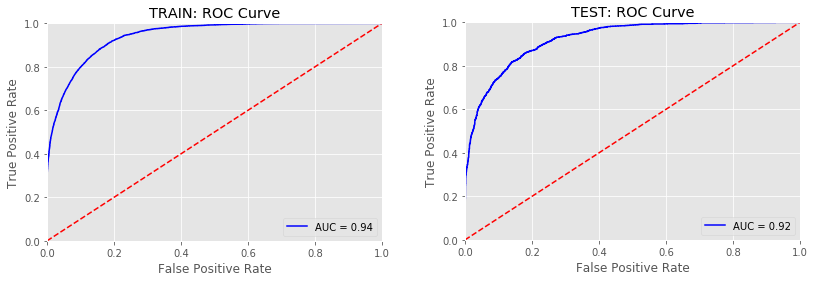Fig. 1: Receiving Operating Characteristic (ROC) curves for Train and Test sets.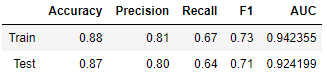Fig. 2: XGBoost performance metrics

The model’s performance seems to be pretty acceptable.

# 3. Global Importance

The techniques used to evaluate the global behavior of the model will be:

3.1 - Feature Importance (evaluated by the XGBoost model and by SHAP)

3.2 - Summary Plot (SHAP)

3.3 - Permutation Importance (ELI5)

3.4 - Partial Dependence Plot (PDPBox and SHAP)

3.5 - Global Surrogate Model (Decision Tree and Logistic Regression)

## 3.1 - Feature Importance

• XGBoost (model-specific)
``````feat_importances = pd.Series(clf_xgb_df.feature_importances_, index=X_train.columns).sort_values(ascending=True)
feat_importances.tail(20).plot(kind='barh')
``````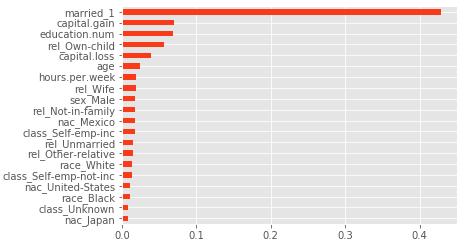Fig. 3: XGBoost Feature Importance

When working with XGBoost, one must be careful when interpreting features importances, since the results might be misleading. This is because the model calculates several importance metrics, with different interpretations. It creates an importance matrix, which is a table with the first column including the names of all the features actually used in the boosted trees, and the other with the resulting ‘importance’ values calculated with different metrics (Gain, Cover, Frequence). A more thourough explanation of these can be found here.

The **Gain **is the most relevant attribute to interpret the relative importance (i.e. improvement in accuracy) of each feature.

• SHAP

In general, SHAP library is considered to be a model-agnostic tool for addressing interpretability (we will cover SHAP’s intuition in the Local Importance section). However, the library has a model-specific method for tree-based machine learning models such as decision trees, random forests and gradient boosted trees.

``````explainer = shap.TreeExplainer(clf_xgb_df)
shap_values = explainer.shap_values(X_test)

shap.summary_plot(shap_values, X_test, plot_type = 'bar')
``````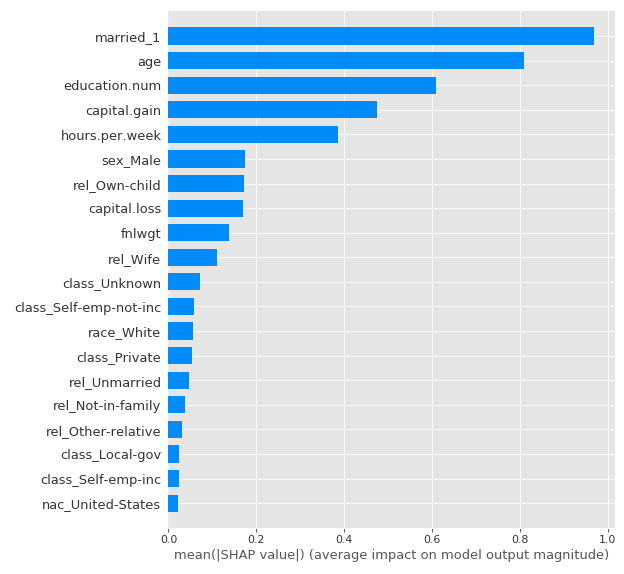Fig. 4: SHAP Feature Importance

The XGBoost feature importance was used to evaluate the relevance of the predictors in the model’s outputs for the Train dataset and the SHAP one to evaluate it for Test dataset, in order to assess if the most important features were similar in both approaches and sets.

It is observed that the most important variables of the model are maintained, although in different order of importance (age seems to take much more relevance in the test set by SHAP approach).

## 3.2 Summary Plot (SHAP)

The SHAP Summary Plot is a very interesting plot to evaluate the features of the model, since it provides more information than the traditional Feature Importance:

• Feature Importance: variables are sorted in descending order of importance.
• Impact on Prediction: the position on the horizontal axis indicates whether the values of the dataset instances for each feature have more or less impact on the output of the model.
• Original Value: the color indicates, for each feature, whether it is a high or low value (in the range of each of the feature).
• Correlation: the correlation of a feature with the model output can be analyzed by evaluating its color (its range of values) and the impact on the horizontal axis. For example, it is observed that the age has a positive correlation with the target, since the impact on the output increases as the value of the feature increases.
``````shap.summary_plot(shap_values, X_test)
``````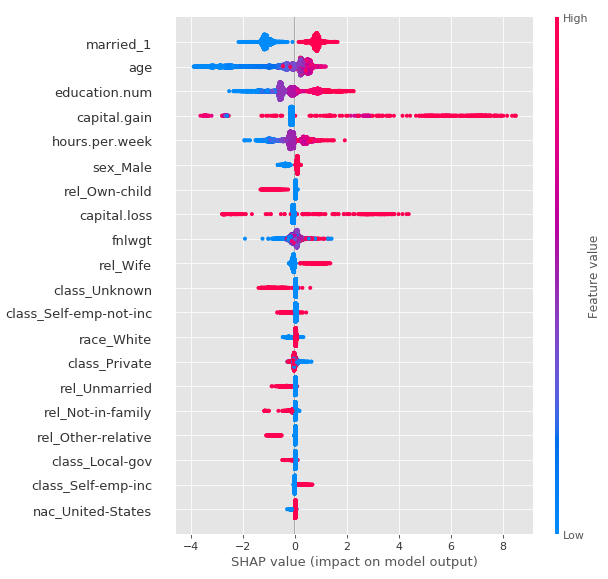Fig. 5: SHAP Summary Plot

## 3.3 - Permutation Importance (ELI5)

Another way to assess the global importance of the predictors is to randomly permute the order of the instances for each feature in the dataset and predict with the trained model. If by doing this disturbance in the order, the evaluation metric does not change substantially, then the feature is not so relevant. If instead the evaluation metric is affected, then the feature is considered important in the model. This process is done individually for each feature.

To evaluate the trained XGBoost model, the Area Under the Curve (AUC) of the ROC Curve will be used as the performance metric. Permutation Importance will be analyzed in both Train and Test:

``````# Train
perm = PermutationImportance(clf_xgb_df, scoring = 'roc_auc', random_state=1984).fit(X_train, y_train)
eli5.show_weights(perm, feature_names = X_train.columns.tolist())

# Test
perm = PermutationImportance(clf_xgb_df, scoring = 'roc_auc', random_state=1984).fit(X_test, y_test)
eli5.show_weights(perm, feature_names = X_test.columns.tolist())
``````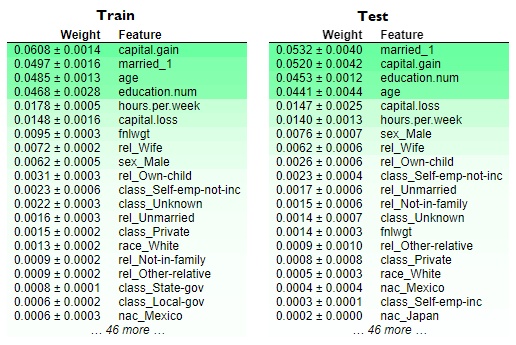Fig. 6: Permutation Importance for Train and Test sets.

Even though the order of the most important features changes, it looks like that the most relevant ones remain the same. It is interesting to note that, unlike the XGBoost Feature Importance, the age variable in the Train set has a fairly strong effect (as showed by SHAP Feature Importance in the Test set). Furthermore, the 6 most important variables according to the Permutation Importance are kept in Train and Test (the difference in order may be due to the distribution of each sample).

The coherence between the different approaches to approximate the global importance generates more confidence in the interpretation of the model’s output.

#model-interpretability #model-fairness #interpretability #machine-learning #shapley-values #deep learning1620754080

## Top 4 Cloud Computing Models Explained

Whether you are a business owner looking to shift your current on-premise infrastructure to the cloud, or a student who wants to start learning cloud computing, the first step is knowing about cloud computing models. The three models that you will come across are – IaaS, PaaS, and SaaS. These models have many distinct features. You can avail of these cloud services over the Internet easily.

### Cloud Computing Models

#### 1. IaaS (Infrastructure as a Service)

IaaS is one of the most important cloud computing models that provides you with networking hardware over the Internet. These resources are provided to you through virtualization. This means that you can log in to an IaaS platform to use virtual machines (VM) to install an OS or software and run databases. This VM can work as a virtual data center.

#cloud computing #cloud computing models #cloud models #cloud1641805837

## How to Predict Housing Prices with Linear Regression?The final objective is to estimate the cost of a certain house in a Boston suburb. In 1970, the Boston Standard Metropolitan Statistical Area provided the information. To examine and modify the data, we will use several techniques such as data pre-processing and feature engineering. After that, we'll apply a statistical model like regression model to anticipate and monitor the real estate market.

Project Outline:

• EDA
• Feature Engineering
• Pick and Train a Model
• Interpret
• Conclusion

### EDA

Before using a statistical model, the EDA is a good step to go through in order to:

• Recognize the data set
• Check to see if any information is missing.
• Find some outliers.
• To get more out of the data, add, alter, or eliminate some features.

### Importing the Libraries

• Recognize the data set
• Check to see if any information is missing.
• Find some outliers.
• To get more out of the data, add, alter, or eliminate some features.

# Import the libraries #Dataframe/Numerical libraries import pandas as pd import numpy as np #Data visualization import plotly.express as px import matplotlib import matplotlib.pyplot as plt import seaborn as sns #Machine learning model from sklearn.linear_model import LinearRegression

### Have a Look at the Columns

Crime: It refers to a town's per capita crime rate.

ZN: It is the percentage of residential land allocated for 25,000 square feet.

Indus: The amount of non-retail business lands per town is referred to as the indus.

CHAS: CHAS denotes whether or not the land is surrounded by a river.

NOX: The NOX stands for nitric oxide content (part per 10m)

RM: The average number of rooms per home is referred to as RM.

AGE: The percentage of owner-occupied housing built before 1940 is referred to as AGE.

DIS: Weighted distance to five Boston employment centers are referred to as dis.

TAX: The TAX columns denote the rate of full-value property taxes per \$10,000 dollars.

B: B=1000(Bk — 0.63)2 is the outcome of the equation, where Bk is the proportion of blacks in each town.

PTRATIO: It refers to the student-to-teacher ratio in each community.

LSTAT: It refers to the population's lower socioeconomic status.

MEDV: It refers to the 1000-dollar median value of owner-occupied residences.

### Data Preprocessing

# Check if there is any missing values. housing_df.isna().sum() CRIM       0 ZN         0 INDUS      0 CHAS       0 NOX        0 RM         0 AGE        0 DIS        0 RAD        0 TAX        0 PTRATIO    0 B          0 LSTAT      0 MEDV       0 dtype: int64

No missing values are found

We examine our data's mean, standard deviation, and percentiles.

housing_df.describe()The crime, area, sector, nitric oxides, 'B' appear to have multiple outliers at first look because the minimum and maximum values are so far apart. In the Age columns, the mean and the Q2(50 percentile) do not match.

We might double-check it by examining the distribution of each column.

### Inferences

1. The rate of crime is rather low. The majority of values are in the range of 0 to 25. With a huge value and a value of zero.
2. The majority of residential land is zoned for less than 25,000 square feet. Land zones larger than 25,000 square feet represent a small portion of the dataset.
3. The percentage of non-retial commercial acres is mostly split between two ranges: 0-13 and 13-23.
4. The majority of the properties are bordered by the river, although a tiny portion of the data is not.
5. The content of nitrite dioxide has been trending lower from.3 to.7, with a little bump towards.8. It is permissible to leave a value in the range of 0.1–1.
6. The number of rooms tends to cluster around the average.
7. With time, the proportion of owner-occupied units rises.
8. As the number of weights grows, the weight distance between 5 employment centers reduces. It could indicate that individuals choose to live in new high-employment areas.
9. People choose to live in places with limited access to roadways (0-10). We have a 30th percentile outlier.
10. The majority of dwelling taxes are in the range of \$200-450, with large outliers around \$700,000.
11. The percentage of people with lower status tends to cluster around the median. The majority of persons are of lower social standing.

Because the model is overly generic, removing all outliers will underfit it. Keeping all outliers causes the model to overfit and become excessively accurate. The data's noise will be learned.

The approach is to establish a happy medium that prevents the model from becoming overly precise. When faced with a new set of data, however, they generalise well.

We'll keep numbers below 600 because there's a huge anomaly in the TAX column around 600.

new_df=housing_df[housing_df['TAX']<600]

### Looking at the DistributionThe overall distribution, particularly the TAX, PTRATIO, and RAD, has improved slightly.

### CorrelationPerfect correlation is denoted by the clear values. The medium correlation between the columns is represented by the reds, while the negative correlation is represented by the black.

With a value of 0.89, we can see that 'MEDV', which is the medium price we wish to anticipate, is substantially connected with the number of rooms 'RM'. The proportion of black people in area 'B' with a value of 0.19 is followed by the residential land 'ZN' with a value of 0.32 and the percentage of black people in area 'ZN' with a value of 0.32.

The metrics that are most connected with price will be plotted.## Feature Engineering

### Feature Scaling

Gradient descent is aided by feature scaling, which ensures that all features are on the same scale. It makes locating the local optimum much easier.

Mean standardization is one strategy to employ. It substitutes (target-mean) for the target to ensure that the feature has a mean of nearly zero.

def standard(X):    '''Standard makes the feature 'X' have a zero mean'''    mu=np.mean(X) #mean    std=np.std(X) #standard deviation    sta=(X-mu)/std # mean normalization    return mu,std,sta     mu,std,sta=standard(X) X=sta X

### Choose and Train the Model

For the sake of the project, we'll apply linear regression.

Typically, we run numerous models and select the best one based on a particular criterion.

Linear regression is a sort of supervised learning model in which the response is continuous, as it relates to machine learning.

Form of Linear Regression

y= θX+θ1 or y= θ1+X1θ2 +X2θ3 + X3θ4

y is the target you will be predicting

0 is the coefficient

x is the input

We will Sklearn to develop and train the model

#Import the libraries to train the model from sklearn.model_selection import train_test_split from sklearn.linear_model import LinearRegression

Allow us to utilise the train/test method to learn a part of the data on one set and predict using another set using the train/test approach.

X_train,X_test,y_train,y_test=train_test_split(X,y,test_size=0.4) #Create and Train the model model=LinearRegression().fit(X_train,y_train) #Generate prediction predictions_test=model.predict(X_test) #Compute loss to evaluate the model coefficient= model.coef_ intercept=model.intercept_ print(coefficient,intercept) [7.22218258] 24.66379606613584

In this example, you will learn the model using below hypothesis:

Price= 24.85 + 7.18* Room

It is interpreted as:

For a decided price of a house:

A 7.18-unit increase in the price is connected with a growth in the number of rooms.

As a side note, this is an association, not a cause!

### Interpretation

You will need a metric to determine whether our hypothesis was right. The RMSE approach will be used.

Root Means Square Error (RMSE) is defined as the square root of the mean of square error. The difference between the true and anticipated numbers called the error. It's popular because it can be expressed in y-units, which is the median price of a home in our scenario.

def rmse(predict,actual):    return np.sqrt(np.mean(np.square(predict - actual))) # Split the Data into train and test set X_train,X_test,y_train,y_test=train_test_split(X,y,test_size=0.4) #Create and Train the model model=LinearRegression().fit(X_train,y_train) #Generate prediction predictions_test=model.predict(X_test) #Compute loss to evaluate the model coefficient= model.coef_ intercept=model.intercept_ print(coefficient,intercept) loss=rmse(predictions_test,y_test) print('loss: ',loss) print(model.score(X_test,y_test)) #accuracy [7.43327725] 24.912055881970886 loss: 3.9673165450580714 0.7552661033654667 Loss will be 3.96

This means that y-units refer to the median value of occupied homes with 1000 dollars.

This will be less by 3960 dollars.

While learning the model you will have a high variance when you divide the data. Coefficient and intercept will vary. It's because when we utilized the train/test approach, we choose a set of data at random to place in either the train or test set. As a result, our theory will change each time the dataset is divided.

This problem can be solved using a technique called cross-validation.

### Improvisation in the Model

With 'Forward Selection,' we'll iterate through each parameter to assist us choose the numbers characteristics to include in our model.

### Forward Selection

1. Choose the most appropriate variable (in our case based on high correlation)
2. Add the next best variable to the model
3. Some predetermined conditions must meet.

We'll use a random state of 1 so that each iteration yields the same outcome.

cols=[] los=[] los_train=[] scor=[] i=0 while i < len(high_corr_var):    cols.append(high_corr_var[i])        # Select inputs variables    X=new_df[cols]        #mean normalization    mu,std,sta=standard(X)    X=sta        # Split the data into training and testing    X_train,X_test,y_train,y_test= train_test_split(X,y,random_state=1)        #fit the model to the training    lnreg=LinearRegression().fit(X_train,y_train)        #make prediction on the training test    prediction_train=lnreg.predict(X_train)        #make prediction on the testing test    prediction=lnreg.predict(X_test)        #compute the loss on train test    loss=rmse(prediction,y_test)    loss_train=rmse(prediction_train,y_train)    los_train.append(loss_train)    los.append(loss)        #compute the score    score=lnreg.score(X_test,y_test)    scor.append(score)        i+=1

We have a big 'loss' with a smaller collection of variables, yet our system will overgeneralize in this scenario. Although we have a reduced 'loss,' we have a large number of variables. However, if the model grows too precise, it may not generalize well to new data.

In order for our model to generalize well with another set of data, we might use 6 or 7 features. The characteristic chosen is descending based on how strong the price correlation is.

high_corr_var ['RM', 'ZN', 'B', 'CHAS', 'RAD', 'DIS', 'CRIM', 'NOX', 'AGE', 'TAX', 'INDUS', 'PTRATIO', 'LSTAT']

With 'RM' having a high price correlation and LSTAT having a negative price correlation.

# Create a list of features names feature_cols=['RM','ZN','B','CHAS','RAD','CRIM','DIS','NOX'] #Select inputs variables X=new_df[feature_cols] # Split the data into training and testing sets X_train,X_test,y_train,y_test= train_test_split(X,y, random_state=1) # feature engineering mu,std,sta=standard(X) X=sta # fit the model to the trainning data lnreg=LinearRegression().fit(X_train,y_train) # make prediction on the testing test prediction=lnreg.predict(X_test) # compute the loss loss=rmse(prediction,y_test) print('loss: ',loss) lnreg.score(X_test,y_test) loss: 3.212659865936143 0.8582338376696363

The test set yielded a loss of 3.21 and an accuracy of 85%.

Other factors, such as alpha, the learning rate at which our model learns, could still be tweaked to improve our model. Alternatively, return to the preprocessing section and working to increase the parameter distribution.

For more details regarding scraping real estate data you can contact Scraping Intelligence today

https://www.websitescraper.com/how-to-predict-housing-prices-with-linear-regression.php1620874140

## Cloud Computing Vs Grid Computing

The similarity between cloud computing and grid computing is uncanny. The underlying concepts that make these two inherently different are actually so similar to one and another, which is responsible for creating a lot of confusion. Both cloud and grid computing aims to provide a similar kind of services to a large user base by sharing assets among an enormous pool of clients.

Both of these technologies are obviously network-based and are capable enough to sport multitasking. The availability of multitasking allows the users of either of the two services to use multiple applications at the same time. You are also not limited to the kind of applications that you can use. You are free to choose any number of applications that can accomplish any tasks that you want. Learn more about cloud computing applications.

#cloud computing #cloud computing vs grid computing #grid computing #cloud